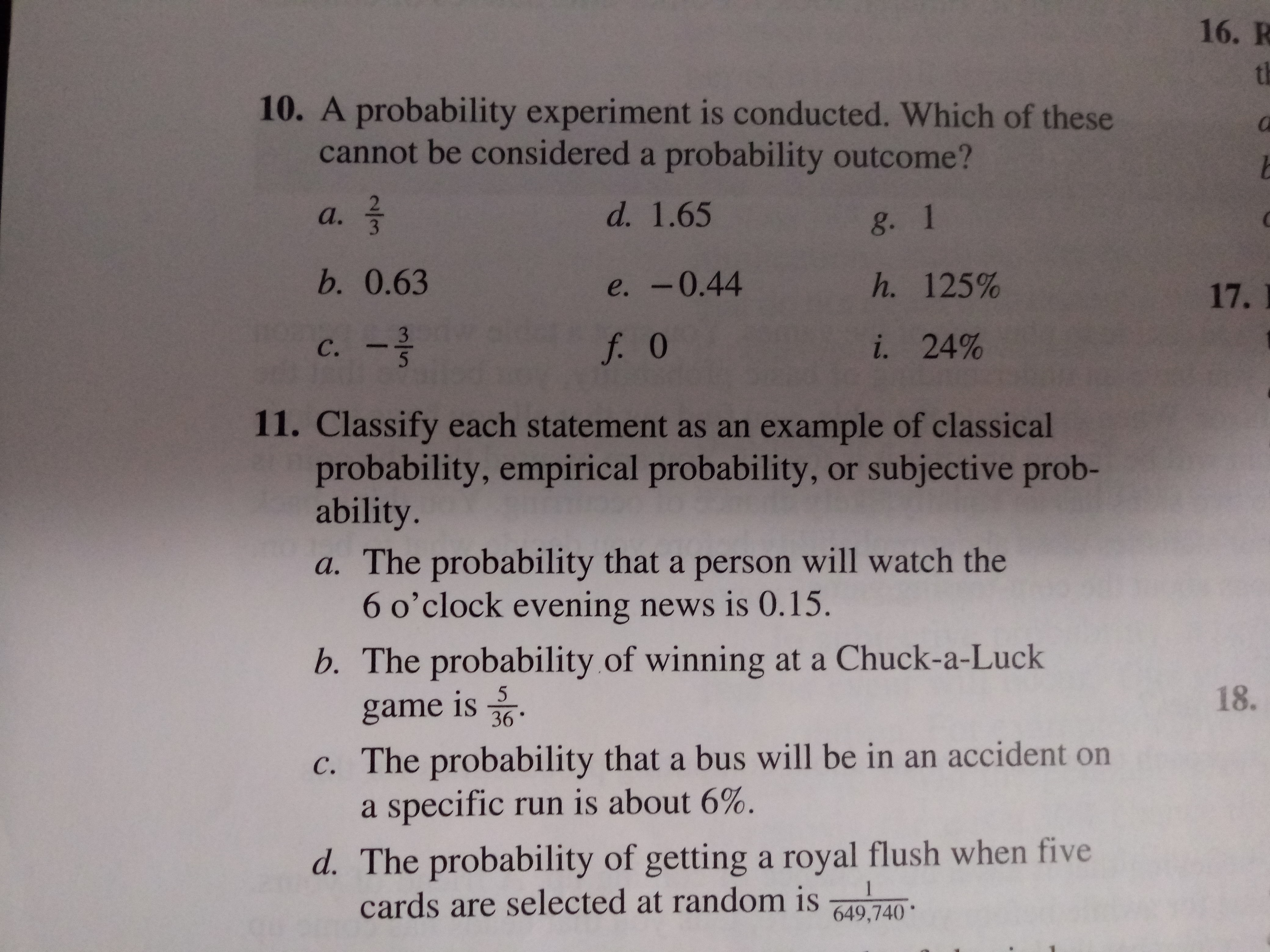# 16. Rth10. A probability experiment is conducted. Which of thesecannot be considered a probability outcome?d. 1.65а.g. 1b. 0.63h. 125%e. -0.4417.3i. 24%f. 0с.11. Classify each statement as an example of classicalprobability, empirical probability, or subjective prob-ability.a. The probability that a person will watch the6 o'clock evening news is O.15.b. The probability of winning at a Chuck-a-Luck18.5game is36c. The probability that a bus will be in an accident ona specific run is about 6%.d. The probability of gettinga royal flush when fivecards are selected at random is1649,740

Questionhelp_outlineImage Transcriptionclose16. R th 10. A probability experiment is conducted. Which of these cannot be considered a probability outcome? d. 1.65 а. g. 1 b. 0.63 h. 125% e. -0.44 17. 3 i. 24% f. 0 с. 11. Classify each statement as an example of classical probability, empirical probability, or subjective prob- ability. a. The probability that a person will watch the 6 o'clock evening news is O.15. b. The probability of winning at a Chuck-a-Luck 18. 5 game is 36 c. The probability that a bus will be in an accident on a specific run is about 6%. d. The probability of gettinga royal flush when five cards are selected at random is 1 649,740 fullscreen
check_circleExpert Solution
Step 1

The probability of an experiment...

### Want to see the full answer?

See Solution

#### Want to see this answer and more?

Solutions are written by subject experts who are available 24/7. Questions are typically answered within 1 hour*

See Solution
*Response times may vary by subject and question
Tagged in

### Basic Probability